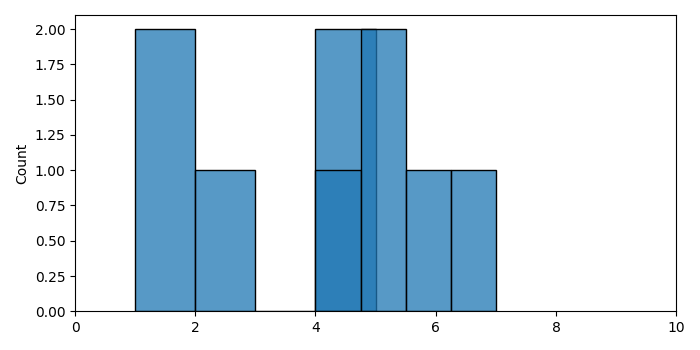# How to plot multiple histograms on same plot with Seaborn using Matplotlib?

To plot multiple histograms on same plot with Seaborn, we can take the following steps −

• Create two lists (x and y).

• Create a figure and add a set of two subplots.

• Iterate a list consisting of x and y.

• Plot a histogram with histplot() method using the data in the list (step 3).

• Limit the X-axis range from 0 to 10.

• To display the figure, use show() method.

## Example

import seaborn as sns
from matplotlib import pyplot as plt
plt.rcParams["figure.figsize"] = [7.00, 3.50]
plt.rcParams["figure.autolayout"] = True
x = [1, 5, 1, 4, 2]
y = [7, 5, 6, 4, 5]
fig, ax = plt.subplots()
for a in [x, y]:
sns.histplot(a, bins=4, ax=ax, kde=False)
ax.set_xlim([0, 10])
plt.show()

## Output